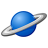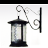# QlikView App Dev

Discussion Board for collaboration related to QlikView App Development.

Announcements
Join this live chat April 6, 10AM EST - QlikView to Qlik Sense REGISTER
cancel
Showing results for
Search instead for
Did you mean:Creator II

## Exporting to Excel in a faster way

Hi all,

I am exporting a table (which exceeds the limit for excel, 1048576 rows) copying the object and pasting cell by cell to the excel.

This operation is taking more than a day to complete and sometimes seems that Qlikview is not responding, just idle.

I've tried using another commands, like copying n' paste and also ExportBiff. In the first it reaches the row limit and the application stops. For the second, excel cannot recognize the object. In this case my question is: is there a way to export objects faster by using a macro.

The code is below:

Sub ExcelOpen(strExcelOpenFile, strExelOpendObjectID)

' Create an instance of Excel

Dim objExcelApp

SET objExcelApp = CREATEOBJECT("Excel.Application")

Int LimitEx

Int intObjectRowCont

' Open workbook

WITH objExcelApp

.DefaultSaveFormat = xlWorkbookNormal

.DisplayAlerts = FALSE

.Workbooks.Open strExcelOpenFile

.DisplayFullScreen = FALSE

.Visible = FALSE

END WITH

' Set worksheet

SET objExcelSheet = objExcelApp.Worksheets(1)

' Clear content

objExcelSheet.Cells.Clear

' Set Excel used range

SET objExcelRange = objExcelSheet.Range("A1048576").End(-4162)

' Last used row in column A

intExcelLastRow = objExcelRange.Row

' Set object to append from

SET objObjectFrom = ActiveDocument.GetSheetObject(strExelOpendObjectID)

' Loop all rows of the object except first header row

FOR intObjectRow = 0 To objObjectFrom.GetRowCount - 1

' Loop all columns of the object

FOR intObjectColumn = 0 To objObjectFrom.GetColumnCount - 1

' Get object data

SET objCell = objObjectFrom.GetCell(intObjectRow, intObjectColumn)

' Add that data to Excel cell

objExcelSheet.Cells(intObjectRow + intExcelLastRow, intObjectColumn + 1) = objCell.Text

NEXT

'add another sheet in case of exceeding limit

IF intObjectRow = 1048576 THEN

objExcelSheet.SaveAs strExcelOpenFile

intObjectRowCont = intObjectRow

intObjectRow = objObjectFrom.GetRowCount +1

LimitEx = 1

END IF

NEXT

IF LimitEx = 1 then

' Set worksheet

SET objExcelSheet = objExcelApp.Worksheets(2)

' Clear content

objExcelSheet.Cells.Clear

' Set Excel used range

SET objExcelRange = objExcelSheet.Range("A1048576").End(-4162)

' Last used row in column A

intExcelLastRoww = objExcelRange.Row

' Loop all rows of the object except first header row

FOR intObjectRow = 0 To objObjectFrom.GetRowCount - 1

' Loop all columns of the object

FOR intObjectColumn = 0 To objObjectFrom.GetColumnCount - 1

' Get object data

SET objCell = objObjectFrom.GetCell(intObjectRow, intObjectColumn)

' Verifying if it is copying the header

IF intObjectRow = 0 THEN

' Add that data to Excel cell

objExcelSheet.Cells(intObjectRow  + intExcelLastRoww, intObjectColumn + 1) = objCell.Text

ELSE

objExcelSheet.Cells(intObjectRow - intObjectRowCont  + intExcelLastRoww, intObjectColumn + 1) = objCell.Text

END IF

NEXT

'Moving for continuing copy

IF intObjectRow = 0 THEN

intObjectRow = intObjectRowCont

END IF

NEXT

END IF

' Save and quit

objExcelSheet.SaveAs strExcelOpenFile

objExcelApp.Application.Quit

SET objExcelSheet = NOTHING

SET objExcelApp = NOTHING

END sub

Sub CallApp (PathName, obj)

DIM xls, Path, Objet

SET fso      = CreateObject("Scripting.FileSystemObject")

IF fso.FileExists(PathName) Then

Call ExcelOpen (PathName, obj)

ELSE

set xls = CreateObject("Excel.Application")

xls.Workbooks.Add

set objsheet = xls.ActiveWorkbook.WorkSheets(1)

xls.ActiveWorkbook.Sheets.Add

' Save and quit

objsheet.SaveAs PathName

xls.ActiveWorkbook.Close

xls.Application.Quit

'Clean

set xls = Nothing

'Call update

Call ExcelOpen (PathName, obj)

End IF

End Sub

Sub MainApp

ActiveDocument.Reload

ActiveDocument.ClearAll True

Call CallApp  ("C:\Test.xlsx" , "TB01")

Application.Save

Application.Quit

End Sub

1 ReplyMaster II

Can't you save a table as csv-file?

Why would you handle such big data via Excel then?

You may also load the file in Access and retrieve the data via Excel as Pivot-Table. This way you may have access to the entire dataset with a lot of Excel-functionalities.